# Multi-dimensional logarithmic residues

(diff) ← Older revision | Latest revision (diff) | Newer revision → (diff)

By a logarithmic residue formula one usually understands an integral representation for the sum of the values of a holomorphic function at all the zeros of a holomorphic mapping in a given domain, where the number of times each zero is taken is equal to the multiplicity of the zero (for instance, a formula for the number of these zeros). Consider a mapping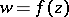(a1)

which is holomorphic on the closed domain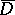and has no zeros on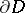, whereis a bounded domain inwith piecewise smooth boundary,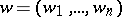,,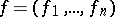. Consider a function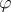holomorphic inand continuous on.

The following assertion is due to G. Roos [a1]: If the vector function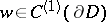is such thaton, then(a2)Here,,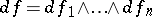,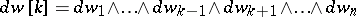, and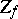is the set of zeros of the mapping (a1) in. The sum at the right-hand side of (a2) can be written as integrals of various dimensions fromto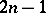[a1] and in terms of currents, and the corresponding integration is over the whole complex manifold (as in the Poincaré–Lelong formula and for the Coleff–Herrera residue current; [a2]).

## Applications.

Applications of multi-dimensional logarithmic residues to series expansion of implicit functions, the computation of the zero-multiplicity of a holomorphic mapping and to the theory of numbers are given in [a1], [a2].

Consider the system of algebraic equations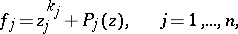(a3)

where the degree of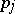is less than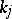for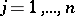.

Ifis a polynomial of degree, then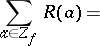(a4)whereis the Jacobian of the system (a3) andis the linear functional acting on the polynomials in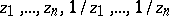by associating to any such polynomial its free term (L. Aizenberg, cf. [a1]).

Using formula (a4) one can compute power sums of, for example, the first coordinates of the roots of the system (a3),whereis the number of roots. The coefficients of the polynomial, having roots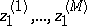, are given by Waring's formula or Newton's recurrence formula. Thus, one has obtained a new method for eliminating unknowns; this method does not add extra roots and does not omit any root. This method appears to be simpler than the classical methods of elimination using the resultants of polynomials. Formula (a4) leads to a particularly simple computation when the degree of the polynomial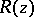is small.

### Example.

Consider in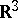the three surfaces of third order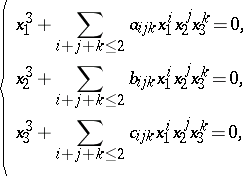(a5)

where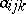,and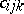are real numbers. Let the surfaces in (a5) be in "general position" in the sense that they have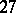points in common in, the maximum possible number. Fix a point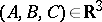and compute, using (a4), the sum of the squares of the distances from this point to thecommon points of the surfaces (a5). This sum is equal to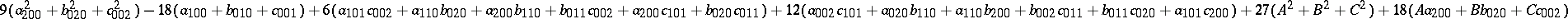. If is curious that the answer does not depend on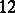of the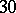coefficients of the equations of the surfaces (a5).

## Generalization.

There exists a more general formula than (a4) for systems of algebraic equations(a6)

where the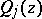are homogeneous polynomials withas their highest degree while the degree of eachis less than,. It is assumed that the only common zero of the polynomialsis the origin (see [a1]). This generalized formula for system (a6) has found application in the determination of all stationary solutions of certain chemical kinetic equations [a3].

How to Cite This Entry:
Multi-dimensional logarithmic residues. Encyclopedia of Mathematics. URL: http://encyclopediaofmath.org/index.php?title=Multi-dimensional_logarithmic_residues&oldid=14334
This article was adapted from an original article by L. Aizenberg (originator), which appeared in Encyclopedia of Mathematics - ISBN 1402006098. See original article